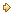Visitors Online: 77 | Thursday 23rd May 2019

CBSE Guess > Papers > Question Papers > Class XII > 2004 > Economics > Compartment Delhi Set-I

ECONOMICS (Set I—Compartment Delhi)

SECTION – A

Q. 1. Answer the following questions: 1x4
(i) Give meaning of opportunity cost.
(ii) Define production function.
(iii) Give meaning of producer’s equilibrium.
(iv) Give one example of variable cost.

Q. 2. Explain the central problem of ‘what to produce’. 3

Q. 3. What is the relation between the change in the price of a good and the change in demand of its substitute good? Explain with the help of an example. 1, 2

Q. 4. How is equilibrium price determined under perfect competition? Explain with the help of a diagram. 3

Q. 5. What happens to equilibrium price when there is decrease in demand? Explain with the help of a diagram. 3

Q. 6. At a price of Rs. 4 per unit a consumer buys 50 units of a good. The price elasticity of demand is —2. How many units will the consumer buy at Rs. 3 per unit? 4

Q. 7. Given that Fixed Cost is Rs. 20, calculate (a) Total Variable Cost and (b) Total Cost from the following: 4

 Output (Unit) Marginal Cost (Rs.) 0 1 2 3 0 10 15 25

Q. 8. Explain the effects on output when all inputs are increased in the same proportion. 4

Q. 9. State any two features of monopolistic competition Draw Average Revenue and Marginal Revenue curves of a firm in a single diagram in this market. 4
Or
State any three features of perfect competition. Also draw Average Revenue curve of the firm in this market.

Q. 10. Explain briefly any three factors which lead to ‘increase in demand’. 6

Q. 11. Explain briefly any three determinants of supply of a good. 6
Or
Explain the Law of Variable Proportions. Also state the reasons behind the law.

Q. 12. What is ‘revenue’ of a firm? Give meaning of Average Revenue and Marginal Revenue. What happens to average revenue when marginal revenue is (i) greater than average revenue, (ii) equal to average and (iii) less than average revenue? 6

SECTION - B

Q. 13. Answer the following questions 1x4
(i) Give meaning of macro economics.
(ii) Give one example of micro economics.
(iii) Define foreign exchange.
(iv) What is Balance of Trade?

Q. 14. Calculate Net Value Added at Factor Cost from the following: 3

 Rs. (Lakhs) (i) Purchases of materials (ii) Depreciation (iii) Sales (iv) Excise tax (v) Opening stock (vi) Intermediate consumption (vii) Closing stock 30 12 200 20 15 48 10

Q. 16. Explain briefly the meaning of involuntary unemployment and full employment. 3

Q. 17. Explain the relation between foreign exchange rate and demand for foreign exchange. 3

Q. 18. Explain the ‘unit of value’ function of money. 4
Or
Explain the ‘standard of deferred payment’ function of money.

Q. 19. Explain the ‘issue of currency’ function of a central bank. 4

Q. 20. Explain revenue receipts in a government budget with appropriate examples. 4

Q. 21. Explain the concept of ‘revenue deficit’ in a government budget. 4

Q. 22. Distinguish between intermediate products and final products. Giving reason, state whether the following are intermediate products or final products: 3, 3
(i) Purchase of equipments for installation in a factory
(ii) Purchase of food items by a hotel
(iii) Purchase of armaments by military

Q. 23. Find out (a) National Income and (b) Gross National Disposable Income from the following data: 4, 2

 Rs. (Crores) (i) Private final consumption expenditure (ii) Net current transfers from the rest of the world (iii) Indirect tax (iv) Net domestic capital formation (v) Government final consumption expenditure (vi) Consumption of fixed capital (depreciation) (vii) Subsidies (viii) Exports (ix) Net factor income from abroad (x) Imports 400 (-) 5 65 120 100 20 5 30 (-) 10 40

Q. 24. Explain the role of taxation and government expenditure in reducing aggregate demand in an economy.6 Or
Explain the role of ‘reserve ratio’ and ‘rate of interest’ in reducing aggregate demand in an economy.

 Economics 2004 Question Papers Class XII Delhi Outside Delhi Compartment Delhi Compartment Outside DelhiSet ISet ISet ISet ISet IISet IISet IISet IISet IIISet III

CBSE 2004 Question Papers Class XII